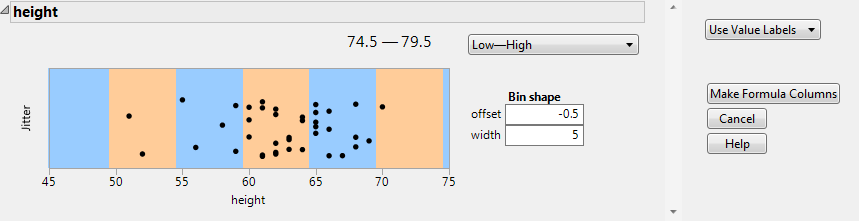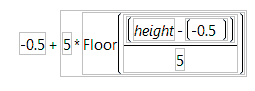Using JMP > Enter and Edit Data > Restructure Data > Make Binning Formula
Publication date: 07/30/2020

## Make Binning Formula

You can distribute your data into equal width bins using the Make Binning Formula option. Select the column or columns that you want to divide into bins, and select Cols > Utilities > Make Binning Formula. New formula columns are added to the data table.

The Make Binning Formula window contains the following options:

Format

Select a format for displaying the range of values in the bin. You can see a preview by moving the cursor over the graph.

Bin Shape: Offset

Select an offset value for the lower edge of the bins.

Note: Bins are identified by their lower edge. The lower edge is in the bin. The upper edge is in the next bin because it is the next bin's lower edge.

Bin Shape: Width

Select the width of values for the bins.

Note: The colored bands reflect the offset and the width of the bins with respect to the data.

Labels

Specify whether value labels are shown instead of the data values.

Use Value Labels

Shows a label instead of the value.

Use Range Labels

Includes the lower and upper values for each range in the label.

No Labels

Uses the lower edge value as the label.

Tip: Value Labels are recommended in most platforms, many of which do not support range labels. In the Categorical platform, you must use value labels. On some axes, you might find that range labels more clearly identify the values, or you can try adjusting the offset and width for the axis.

Make All Like X

(Appears only if multiple columns are selected.) Applies the choices made for the first column (X) to the remaining columns.

Make Formula Columns

Creates the formula columns and closes the window.

#### Example of Making a Binning Formula

1. Select Help > Sample Data Library and open Big Class.jmp.

2. Select the height column.

3. Select Cols > Utilities > Make Binning Formula.

You want the range of values to appear as X-X, so keep the range set to Low - High.

4. Change the offset to -0.5.

Tip: For integer data, setting the offset to -0.5 helps disambiguate values on the edge. In this example, one of the bins covers 59.5 to 64.5, so it is clear that 59 and 65 are not included in this bin.

5. Keep the width set to 5.

6. For the labels, keep it set to Use Value Labels, so that you can see the range of values for the bin.

Figure 4.36 Completed Binning Window7. Click Make Formula Columns.

A column called height Binned is added to the Big Class.jmp data table.

8. To see how the formula is calculated, right-click the height Binned column and select Formula.

Figure 4.37 Formula